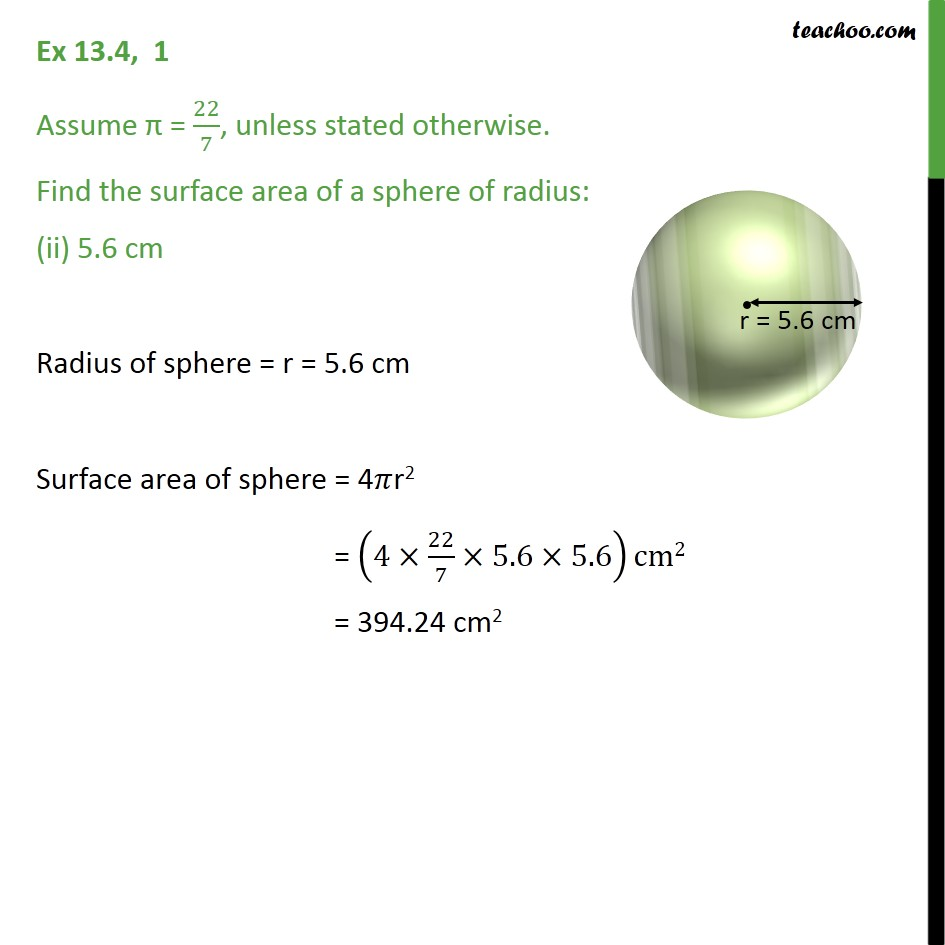1. Chapter 13 Class 9 Surface Areas and Volumes (Term 2)
2. Serial order wise
3. Ex 13.4

Transcript

Ex 13.4, 1 Assume = 22/7, unless stated otherwise. Find the surface area of a sphere of radius: (ii) 5.6 cm Radius of sphere = r = 5.6 cm Surface area of sphere = 4 r2 = (4 22/7 5.6 5.6)cm2 = 394.24 cm2